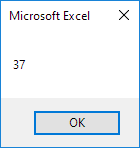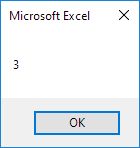# DateDiff Function

The DateDiff function in Excel VBA can be used to get the number of days, weeks, months or years between two dates. You can also use the DateDiff function to calculate the time difference between two times.

### Dates

Place a command button on your worksheet and add the following code lines:

Dim firstDate As Date, secondDate As Date, n As Integer

firstDate = DateValue("Jan 19, 2020")
secondDate = DateValue("Feb 25, 2020")

n = DateDiff("d", firstDate, secondDate)

MsgBox n

Explanation: first, we declare two dates. Next, we initialize the two dates using the DateValue function. The DateDiff function has three arguments. Fill in "d" for the first argument to get the number of days between two dates. Finally, we use a MsgBox to display the number of days between the two dates.

Result when you click the command button on the sheet:Note: use "ww" to get the number of weeks between two dates. Use "m" to get the number of months between two dates. Use "yyyy" to get the number of years between two dates.

### Times

Place a command button on your worksheet and add the following code lines:

Dim firstTime As Date, secondTime As Date, x As Integer

firstTime = TimeValue("2:15:00 pm")
secondTime = TimeValue("5:15:00 pm")

x = DateDiff("h", firstTime, secondTime)

MsgBox x

Explanation: first, we declare two times (times are declared as dates). Next, we initialize the two times using the TimeValue function. The DateDiff function has three arguments. Fill in "h" for the first argument to calculate the number of hours between two times. Finally, we use a MsgBox to display the number of hours between the two times.

Result when you click the command button on the sheet:Note: use "n" to calculate the number of minutes between two times. Use "s" to calculate the number of seconds between two times. Place your cursor on DateDiff in the Visual Basic Editor and click F1 for help on the other interval specifiers.

Go to Next Chapter: Events